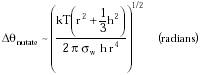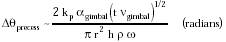Nanomedicine, Volume I: Basic Capabilities

Robert A. Freitas Jr., Nanomedicine, Volume I: Basic Capabilities, Landes Bioscience, Georgetown, TX, 1999

4.3.4.1 Gimballed Nanogyroscopes

Pivoted gyroscopes of the type described in Section 4.3.3.4 are impractical for orientational sensing in all but the largest medical nanodevices. Setting wmax (Eqn. 4.17) equal to wmin (Eqn. 4.18), taking amin = g = 9.81 m/sec2 (gravitational acceleration), and optimizing for minimum sensor size gives a critical nonwobbling nonbursting spin velocity wcrit ~ 6.8 x 107 radians/sec at a minimum device radius of ~35 microns. If a pivoted gyroscope is smaller than this minimum radius, then spinning it slower than wcrit causes the gyro to tumble helplessly, while spinning it faster than wcrit tears the gyro apart.

However, a triaxially gimballed nanogyroscope may be regarded as having its pivot point near its center of mass, so Lp becomes very small and wmin becomes very slow, making nanogyroscopes feasible in medical nanorobots. Taking h = 1 micron, r = 0.5 micron, r = 3510 kg/m3 and amin = g in Eqn. 4.18, then if the gimbals are aligned such that Lp = 1 nm between the pivot point and the effective center of gravity along the rotation axis, then wmin ~ 400 rad/sec. If gimbal tolerances are improved to Lp = 0.1 nm, then wmin ~ 125 rad/sec.

How stable is a nanogyroscopic orientation standard? There are two principal considerations.

First, Brownian thermal rotation in all three angular degrees of freedom (q, j and y) gives rise to a small nutation around the nanogyroscopic precession axis of magnitude Dqnutate ~ pj / py, where pj and py are angular momenta of the gyro.448 The minimum value for pj is approximated by (1/2) kT ~ (1/2) pj2 / I1, where I1 = (1/4) m r2 + (1/12) m h2, the moment of inertia around j, and m = p r2 h r. The maximum value for py is given by the burst-strength condition w < wmax (Eqn. 4.17) as py ~ I3 w, where I3 = (1/2) m r2, the moment of inertia around y. Hence:{Eqn. 4.20}

For T = 310 K, r = 0.5 micron, h = 1 micron, sw ~ 1010 N/m2, Dqnutate ~ 0.8 microradian.

Second, the gimbal bearings have small frictional losses, exerting small torques on the gyro and causing a small precession away from the original orientation. The angular velocity of precession wprecess ~ Tgimbal / (I3 w) (rad/sec) where Tgimbal is the frictional torque caused by an imperfect gimbal bearing. Assume that the gimbal is driven by external forces to oscillate at some frequency ngimbal, and that a gimbal bearing dissipates power at the rate of Pgimbal (watts), so that each oscillatory motion of the gimbal dissipates Egimbal = Pgimbal/ngimbal (joules). If the angular amplitude of the gimbal motion is agimbal (radians) and the gimbal radius is rgimbal, then the gimbal bearing travels Xgimbal = agimbal rgimbal (meters) per gimbal oscillation and the force applied in dissipating an energy Egimbal is Fgimbal = Egimbal/Xgimbal. Thus, the torque applied by each gimbal oscillation event is Tgimbal = Fgimbal rgimbal = Pgimbal / (ngimbal agimbal); Pgimbal = kp vgimbal2 (see Drexler10), where vgimbal = agimbal ngimbal rgimbal is the sliding speed of the gimbal bearing surfaces during movement and kp is a constant that depends solely on the geometry of the bearing.

The change in orientation angle caused by each gimbal oscillation event is Dqosc = wprecess / ngimbal. The number of gimbal oscillations N = t ngimbal, where t is elapsed time since the gyro was last calibrated. If gimbal oscillations are independent and randomly distributed, then the total change in orientation angle is very roughly Dqprecess ~ Dqosc N1/2; assuming rgimbal ~ r, then:{Eqn. 4.21}

From Drexler10, kp = 2.7 x 10-14 watt-sec2/m2 as a conservative value for a small (~2 nm) stiff bearing. Random hydrodynamic flows induced by thermal fluctuations inside biological cells have a characteristic duration tfluct ~ 10 millisec,1069 which suggests agimbal ~ 0.06 radians (~3°) from Eqn. 3.2 for an R ~ 1 micron in cyto nanorobot. Taking r = 0.5 micron, h = 1 micron, r = 3510 kg/m3 for diamond (giving m ~ 3 x 10-15 kg), w = 1 x 109 rad/sec (just below the wmax ~ 4.8 x 109 rad/sec bursting speed), and assuming ngimbal ~ tfluct­1 = 100 Hz (to which the results are not terribly sensitive), then Dqprecess ~ 10-8 t1/2 (radians). Thus a Dqprecess ~ 1 microradian orientation shift takes ~1 hour; a Dqprecess ~ 4 microradian shift takes ~1 day; and a Dqprecess ~ 70 microradian (~10 arcsec) shift takes ~1 year. (Total angular error Dqtotal = Dqprecess + Dqnutate.) Thus a gimballed nanogyroscope may be sufficiently stable to serve as a readily storable, easily transportable, and highly accurate onboard orientation standard.

Last updated on 17 February 2003